What's algebra about? When learning about variables ($x, y, z$), they seem to "hide" a number: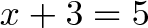$\displaystyle{x + 3 = 5}$

What number could be hiding inside of $x$? 2, in this case.

It seems that arithmetic still works, even when we don't have the exact numbers up front. Later on, we might arrange these "hidden numbers" in complex ways: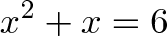$\displaystyle{x^2 + x = 6}$

Whoa -- a bit harder to solve, but it's possible. Today let's figure out how factoring works and why it's useful.

Polynomials

When we write a polynomial like $x^2 + x = 6$, we can think at a higher level.

We have an unknown number, $x$, which interacts with itself ($x * x = x^2$). We add in the original number ($+ x$) and the result is 6.

$x^2$, $x$ and 6 are all "numbers", but now we're keeping track of how they're made:

• $x^2$ is a component interacting with itself
• $x$ is a component on its own
• 6 is the desired state we want the entire system to become

After the interactions are finished, we should get 6. What number could be hiding inside of $x$ to make this true?

Hrm -- this is tricky. So let's fight with a trick of our own: we can make a different system to track the error in our original one (this is mind-bending, so hang on).

Our original system is $x^2 + x$. The desired state is 6. A new system: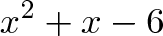$\displaystyle{x^2 + x - 6}$

will track the difference between the original system and the desired state. When are we happiest? When there's no difference: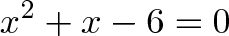$\displaystyle{x^2 + x - 6 = 0}$

Ah! that's why we're so interested in setting polynomials to zero! If we have a system and the desired state, we can make a new equation to track the difference -- and try make it zero. (This is deeper than just "subtract 6 from both sides" -- we're trying to describe the error!)

But... how do we actually get the error to zero? It's still a jumble of components: $x^2$, $x$ and 6 are flying everywhere.

Factor That Mamma Jamma

Factoring the rescue. My intuition: factoring lets us re-arrange a complex system ($x^2 + x - 6$) as a bunch of linked, smaller systems.

Imagine taking a pile of sticks (our messy, disorganized system) and standing them up so they support each other, like a teepee:

/\

(That's a 2-d example, with two sticks).

Remove any stick and the entire structure collapses. If we can rewrite our system:$\displaystyle{x^2 + x - 6 = 0}$

as a series of multiplications:$\displaystyle{\text{Component A} \cdot \text{Component B} = 0}$

we've put the sticks in a "teepee". If Component A or Component B becomes 0, the structure collapses, and we get 0 as a result.

Neat! That is why factoring rocks: we re-arrange our error-system into a fragile teepee, so we can break it. We'll find what obliterates our errors and puts our system in the ideal state.

Remember: We're breaking the error in the system, not the system itself.

Onto The Factoring

Learning to "factor an equation" is the process of arranging your teepee. In this case: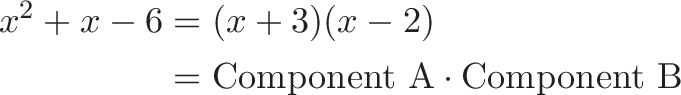\begin{aligned} x^2 + x - 6 &= (x + 3)(x -2) \\ &= \text{Component A} \cdot \text{Component B} \end{aligned}

If $x = -3$ then Component A falls down. If $x = 2$, Component B falls down. Either value causes the error to collapse, which means our original system ($x^2 + x$, the one we almost forgot about!) meets our requirements:

• When $x = -3$, the error collapses, and we get $(-3)^2 + -3 = 6$
• When $x = 2$, the error collapses, and we get $2^2 + 2 = 6$

Putting It All Together

I've wondered about the real purpose of factoring for a long, long time. In algebra class, equations are conveniently set to zero, and we're not sure why. Here's what happens in the real world:

• Define the model: Write how your system behaves ($x^2 + x$)
• Define the desired state: What should it equal? (6)
• Define the error: The error is its own system: Error = actual - desired (i.e., $x^2 + x - 6$)
• Factor the error: Rewrite the error as interlocking components: $(x + 3)(x - 2)$
• Reduce the error to zero: Zero out one component or the other ($x = -3$, or $x = 2$).

When error = 0, our system must be in the desired state. We're done!

Algebra is pretty darn useful:

• Our system is a trajectory, the "desired state" is the target. What trajectory hits the target?
• Our system is our widget sales, the "desired state" is our revenue target. What amount of earnings hits the goal?
• Our system is the probability of our game winning, the "desired state" is a 50-50 (fair) outcome. What settings make it a fair game?

The idea of "matching a system to its desired state" is just one interpretation of why factoring is useful. If you have more, I'd like to hear them!

Appendix

A cheatsheet for the process: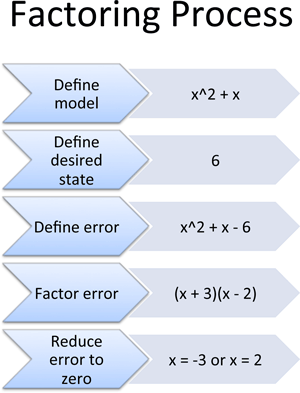Some more food for thought:

• Multiplication is often seen as AND. Component A must be there AND Component B must be there. If either condition is false, the system breaks.

• The Fundamental Theorem of Algebra proves you have as many "components" as the highest polynomial. If your highest term is $x^4$, then you can factor into 4 interlocked components (discussion for another day). But this should make sense: if you rewrite an "$x^4$ system" into multiplications, shouldn't there be 4 individual "$x$ components" being multiplied? If there were 3, you could never get to $x^4$, and if there were 5, you'd overshoot and get an $x^5$ term.

• Do you have a real-world system in a "teepee" arrangement, where a single failing component collapses the entire structure?

• The quadratic formula can "autobreak" any system with $x^2$, $x$ and constant components. There's formulas for complex systems (with $x^3$, $x^4$, or even some $x^5$ components) but they start to get a bit crazy.

• Is there any way to prevent a system from having these weak points? (Unfactorable? Non-zeroable?). Don't forget, we thought systems like $x^2 + 1$ were "non-zeroable" until imaginary numbers came along.

Happy math.